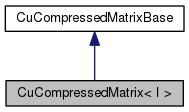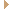CuCompressedMatrix< I > Class Template Reference

Class CuCompressedMatrix, templated on an integer type (expected to be one of: int8, uint8, int16, uint16), this provides a way to approximate a CuMatrix in a more memory-efficient format. More...

`#include <cu-compressed-matrix.h>`

Inheritance diagram for CuCompressedMatrix< I >:[legend]
Collaboration diagram for CuCompressedMatrix< I >:[legend]

## Public Member Functions

CuCompressedMatrix (BaseFloat range, bool truncate=true)
Constructor which sets 'scale_' according to scale_ = range / std::numeric_limits<I>::max(). More...

virtual void CopyFromMat (const CuMatrixBase< BaseFloat > &mat)
Sets *this to an appropriately compressed copy of 'mat', which includes resizing *this. More...

virtual void CopyToMat (CuMatrixBase< BaseFloat > *mat) const
Copies the contents of *this to 'mat', which should be correctly sized beforehand. More...

virtual MatrixIndexT NumRows () const

virtual MatrixIndexT NumCols () const

virtual ~CuCompressedMatrix ()Public Member Functions inherited from CuCompressedMatrixBase
virtual ~CuCompressedMatrixBase ()

void Destroy ()

## Private Attributes

I * data_

BaseFloat scale_

bool truncate_

MatrixIndexT num_rows_

MatrixIndexT num_cols_

MatrixIndexT stride_

## Detailed Description

### template<typename I> class kaldi::CuCompressedMatrix< I >

Class CuCompressedMatrix, templated on an integer type (expected to be one of: int8, uint8, int16, uint16), this provides a way to approximate a CuMatrix in a more memory-efficient format.

It's used in nnet3 to reduce memory use for large networks.

It is *not* a CUDA equivalent for class CompressedMatrix (of ../matrix/compressed-matrix.h). Note: this class is only to be used when you are using a GPU. If you didn't compile for CUDA or you are not using a GPU, you are not supposed to create an instance of this class, and doing so will cause a runtime error.

Definition at line 72 of file cu-compressed-matrix.h.

## ◆ CuCompressedMatrix()

 CuCompressedMatrix ( BaseFloat range, bool truncate = `true` )

Constructor which sets 'scale_' according to scale_ = range / std::numeric_limits<I>::max().

range = 0 (only supported for I == int8) is a special case in which only the sign of the input is retained; and when we reconstruct, the output will be -1, 0 or 1.

truncate (only relevant if range != 0) should be true if it's possible that the input could exceed the allowed input range, i.e. [0, range] if I is unsigned, and [-range, range] if I is signed; and it may be false if you know that the input (the matrix given to CopyFromMat) will have elements only in the allowed range. Setting 'truncate' to false allows the compression code to avoid the bounds check.

Definition at line 38 of file cu-compressed-matrix.cc.

References KALDI_ASSERT, and KALDI_ERR.

38  :
39  data_(NULL), scale_(range / std::numeric_limits<I>::max()),
40  truncate_(truncate), num_rows_(0), num_cols_(0), stride_(0) {
41 #if HAVE_CUDA == 1
42  KALDI_ASSERT(CuDevice::Instantiate().Enabled());
43 #else
44  KALDI_ERR << "You instantiated CuCompressedMatrix while GPU use "
45  "was not compiled in.";
46 #endif
47 }
#define KALDI_ERR
Definition: kaldi-error.h:147
#define KALDI_ASSERT(cond)
Definition: kaldi-error.h:185

## ◆ ~CuCompressedMatrix()

 virtual ~CuCompressedMatrix ( )
inlinevirtual

Definition at line 99 of file cu-compressed-matrix.h.

## ◆ CopyFromMat()

 void CopyFromMat ( const CuMatrixBase< BaseFloat > & mat )
virtual

Sets *this to an appropriately compressed copy of 'mat', which includes resizing *this.

The details of how this is done will be different in different child classes.

Implements CuCompressedMatrixBase.

Definition at line 65 of file cu-compressed-matrix.cc.

66  {
67 #if HAVE_CUDA == 1
68  KALDI_ASSERT(CuDevice::Instantiate().Enabled());
69  if (mat.NumRows() == 0)
70  return;
71  if (num_rows_ != mat.NumRows() || num_cols_ != mat.NumCols()) {
72  Destroy();
73  num_rows_ = mat.NumRows();
74  num_cols_ = mat.NumCols();
75  data_ = static_cast<I*>(
76  CuDevice::Instantiate().Malloc(sizeof(I) * num_rows_ * num_cols_));
78  }
79
80  {
81  CuTimer tim;
82  dim3 dimGrid, dimBlock;
83  GetBlockSizesForSimpleMatrixOperation(NumRows(), NumCols(),
84  &dimGrid, &dimBlock);
85
86  if (scale_ == 0.0) { // scale == 0 calls a different kernel from the others.
87  cuda_mat_compress_sign(dimGrid, dimBlock, mat.Data(), mat.Dim(),
88  data_, stride_);
89  } else {
90  cuda_mat_compress(dimGrid, dimBlock, mat.Data(), mat.Dim(),
91  data_, stride_, float(1.0 / scale_),
92  truncate_);
93  }
94  CU_SAFE_CALL(cudaGetLastError());
95
96  CuDevice::Instantiate().AccuProfile(__func__, tim);
97  }
98 #endif
99 }
virtual MatrixIndexT NumRows() const
virtual MatrixIndexT NumCols() const
#define KALDI_ASSERT(cond)
Definition: kaldi-error.h:185

## ◆ CopyToMat()

 void CopyToMat ( CuMatrixBase< BaseFloat > * mat ) const
virtual

Copies the contents of *this to 'mat', which should be correctly sized beforehand.

Implements CuCompressedMatrixBase.

Definition at line 102 of file cu-compressed-matrix.cc.

102  {
103 #if HAVE_CUDA == 1
104  KALDI_ASSERT(CuDevice::Instantiate().Enabled());
105  KALDI_ASSERT(mat->NumRows() == num_rows_ && mat->NumCols() == num_cols_);
106  {
107  CuTimer tim;
108  dim3 dimGrid, dimBlock;
109  GetBlockSizesForSimpleMatrixOperation(NumRows(), NumCols(),
110  &dimGrid, &dimBlock);
111  BaseFloat scale = (scale_ == 0.0 ? 1.0 : scale_);
112  cuda_mat_uncompress(dimGrid, dimBlock, mat->Data(), mat->Dim(),
113  data_, stride_, float(scale));
114  }
115 #endif
116 }
virtual MatrixIndexT NumRows() const
float BaseFloat
Definition: kaldi-types.h:29
virtual MatrixIndexT NumCols() const
#define KALDI_ASSERT(cond)
Definition: kaldi-error.h:185

## ◆ Destroy()

 void Destroy ( )
private

Definition at line 50 of file cu-compressed-matrix.cc.

Referenced by CuCompressedMatrix< I >::CopyFromMat().

50  {
51 #if HAVE_CUDA == 1
52  if (data_ != NULL) {
53  // we don't bother timing this because Free() won't normally have to
54  // access the GPU at all (due to caching).
55  CuDevice::Instantiate().Free(data_);
56  data_ = NULL;
57  num_rows_ = 0;
58  num_cols_ = 0;
59  stride_ = 0;
60  }
61 #endif
62 }

## ◆ NumCols()

 virtual MatrixIndexT NumCols ( ) const
inlinevirtual

## ◆ NumRows()

 virtual MatrixIndexT NumRows ( ) const
inlinevirtual

## ◆ data_

 I* data_
private

Definition at line 106 of file cu-compressed-matrix.h.

## ◆ num_cols_

 MatrixIndexT num_cols_
private

Definition at line 129 of file cu-compressed-matrix.h.

## ◆ num_rows_

 MatrixIndexT num_rows_
private

Definition at line 128 of file cu-compressed-matrix.h.

## ◆ scale_

 BaseFloat scale_
private

## ◆ stride_

 MatrixIndexT stride_
private

Definition at line 132 of file cu-compressed-matrix.h.

## ◆ truncate_

 bool truncate_
private

Definition at line 126 of file cu-compressed-matrix.h.

Referenced by CuCompressedMatrix< I >::CopyFromMat().

The documentation for this class was generated from the following files: